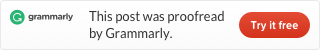Categories

# LeetCode 347. Top K Frequent Elements

Source: https://leetcode.com/problems/top-k-frequent-elements/

Given a non-empty array of integers, return the k most frequent elements.

Example 1:

```Input: nums = [1,1,1,2,2,3], k = 2
Output: [1,2]
```

Example 2:

```Input: nums = , k = 1
Output: ```

Note:

• You may assume k is always valid, 1 ≤ k ≤ number of unique elements.
• Your algorithm’s time complexity must be better than O(n log n), where n is the array’s size.

We use a hashmap to count every number. Then we transform this hashmap to a list, and sort it, output the first k elements.

To see more hashtable related problems, see
Problems and Solutions of Hashmap.This site uses Akismet to reduce spam. Learn how your comment data is processed.